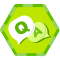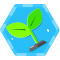# Swift中的异步编程方式

## 引

Swift从代码层面提供了结构化的方式来支持异步编程，在Swift5.5中引入了async和await相关的关键字。需要注意，异步和并行本身是两个概念，在Swift中，异步编程模型已经建立在线程调度之上，这也就是说，我们无需关心其中线程的调用，异步的函数本身就是在子线程中并行执行的，线程切换和调度全有语言本身控制。但是Swift不会保证函数会在哪个特定的线程上执行。

## 异步函数

``````func test(callback: @escaping (_ success: Bool)->Void) {
DispatchQueue.global().async {
callback(true)
}
}
test { success in
print("EndTest")
}
``````

``````Begin <_NSMainThread: 0x600002310100>{number = 1, name = main}
End <_NSMainThread: 0x600002310100>{number = 1, name = main}
Test任务完成 <NSThread: 0x600002300300>{number = 5, name = (null)}
EndTest
``````

``````func test1() async -> Bool {
return true
}

func test2() async -> Bool {
return true
}

async let a = test1()
async let b = test2()
``````

1. 执行完成

2. 抛出异常

3. 永不返回

``````Begin <_NSMainThread: 0x60000329c3c0>{number = 1, name = main}
End <_NSMainThread: 0x60000329c3c0>{number = 1, name = main}
ts1 <NSThread: 0x60000328cc40>{number = 4, name = (null)}
ts2 <NSThread: 0x600003282d40>{number = 6, name = (null)}
``````

``````func test1() async -> Bool {
return true
}

func test2() async -> Bool {
return true
}

let a = await test1()
let b = await test2()
``````

``````Begin <_NSMainThread: 0x600002180140>{number = 1, name = main}
ts1 <NSThread: 0x600002198100>{number = 6, name = (null)}
ts2 <NSThread: 0x6000021accc0>{number = 8, name = (null)}
End <_NSMainThread: 0x600002180140>{number = 1, name = main}
``````

1.异步的方法，属性或函数中。

2.main代码块中。

3.非结构化的子任务代码块中。

``````func test1() async -> Bool {
return true
}

func test2() async -> Bool {
return true
}

async let a = test1()
async let b = test2()
let res = await [a ,b]
print(res)
``````

## 异步序列

Swift中的迭代也支持异步返回，通过AsyncIteratorProtocol协议来定义异步的迭代器，示例如下：

``````struct Group: AsyncSequence {
typealias Element = Int

let limit: Int

struct AsyncIterator : AsyncIteratorProtocol {
let limit: Int
var current = 1
mutating func next() async -> Int? {
return nil
}

guard current <= limit else {
return nil
}

let result = current
current += 1
return result
}
}

func makeAsyncIterator() -> AsyncIterator {
return AsyncIterator(limit: limit)
}
}
print("Begin")
let group = Group(limit: 10)

for await i in group {
print(i)
}
print("End")
``````

## 任务组与任务

``````let res = await withTaskGroup(of: Bool.self, returning: [Bool].self, body: { taskGroup in
let a = await test1()
return a
}
let b = await test1()
return b
}
var datas:[Bool] = []
for await data in taskGroup {
datas.append(data)
}
return datas
})

print(res)
``````

``````class ViewController: UIViewController {

await test()
self.view.backgroundColor = .red
print("Finish")
}
print("Continue")
}

func test() async {
}

override func touchesBegan(_ touches: Set<UITouch>, with event: UIEvent?) {
print("touch")
}
}
``````

``````override func viewDidLoad() {
await test()
self.view.backgroundColor = .red
print("Finish")
return "result"
}
print("Continue")

// 取消任务
}
``````### 珲少etcd 与 Consul 的一致性读对比

43分钟前
2
0

52分钟前
2
0

2
0

100
0

5
0

{{formatHtml(o.title)}}

{{i}}-{{formatHtml(o.content)}}

{{o.author.name}}
{{o.pubDate | formatDate}}
{{o.viewCount | bigNumberTransform}}